# Manhattan plot

What is Manhattan plot?

• Manhattan plot used to visualize the association of SNPs with given trait or disease as statistical significance in terms of P-values on a genomic scale
• In the Manhattan plot, X- and Y-axis represents the SNPs on the chromosomes and associated P-values as −log10[P].
• It is a good way to visualize thousands to millions of SNPs o genome-scale. The lowest the P-value (higher −log10[P]), highest is the association of a given SNP with trait or disease

How to create Manhattan plot?

• We will use `bioinfokit v0.9.2` or later
• Check bioinfokit documentation for installation and documentation
• For generating Manhattan plot, I have used simulated GWAS data for 20K SNPs distributed over 10 chromosomes. Here’s you can download GWAS dataset used for generating Manhattan plot: dataset

Useful reading: Data handling using pandas

``````# you can use interactive python interpreter, jupyter notebook, spyder or python code
# I am using interactive python interpreter (Python 3.7)
>>> from bioinfokit import analys, visuz
# load dataset as pandas dataframe
>>> df = analys.get_data('mhat').data
SNP    pvalue  chr
0  rs0  0.773739    3
1  rs1  0.554637    6
2  rs2  0.733446   10
3  rs3  0.872185    8
4  rs4  0.919034   10

# create Manhattan plot with default parameters
>>> visuz.marker.mhat(df=df, chr='chr',pv='pvalue')
# set parameter show=True, if you want view the image instead of saving
``````

Generated Manhattan plot,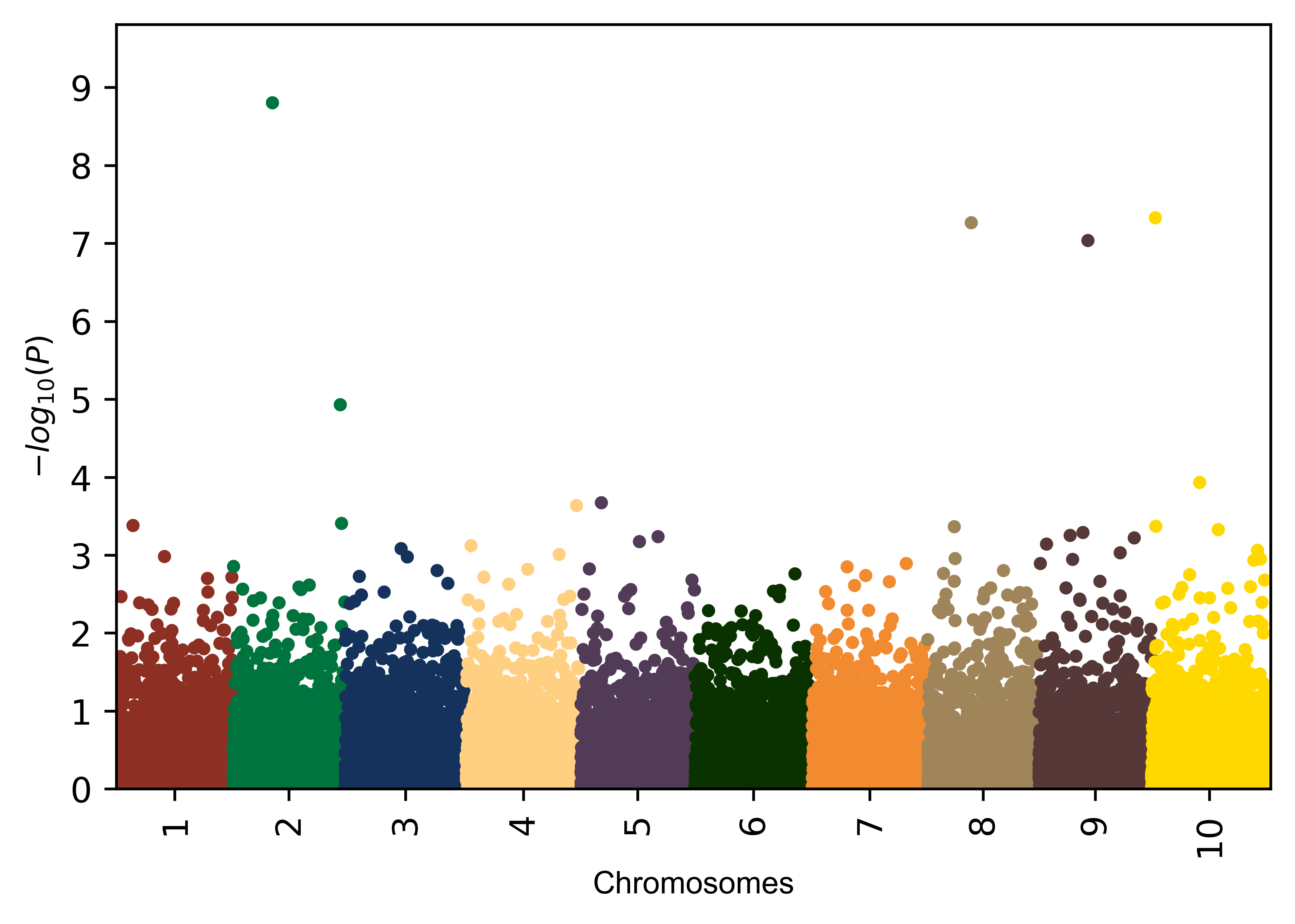Change colors

``````# add alternate two colors
>>> visuz.marker.mhat(df=df, chr='chr',pv='pvalue', color=("#d7d1c9", "#696464"))
``````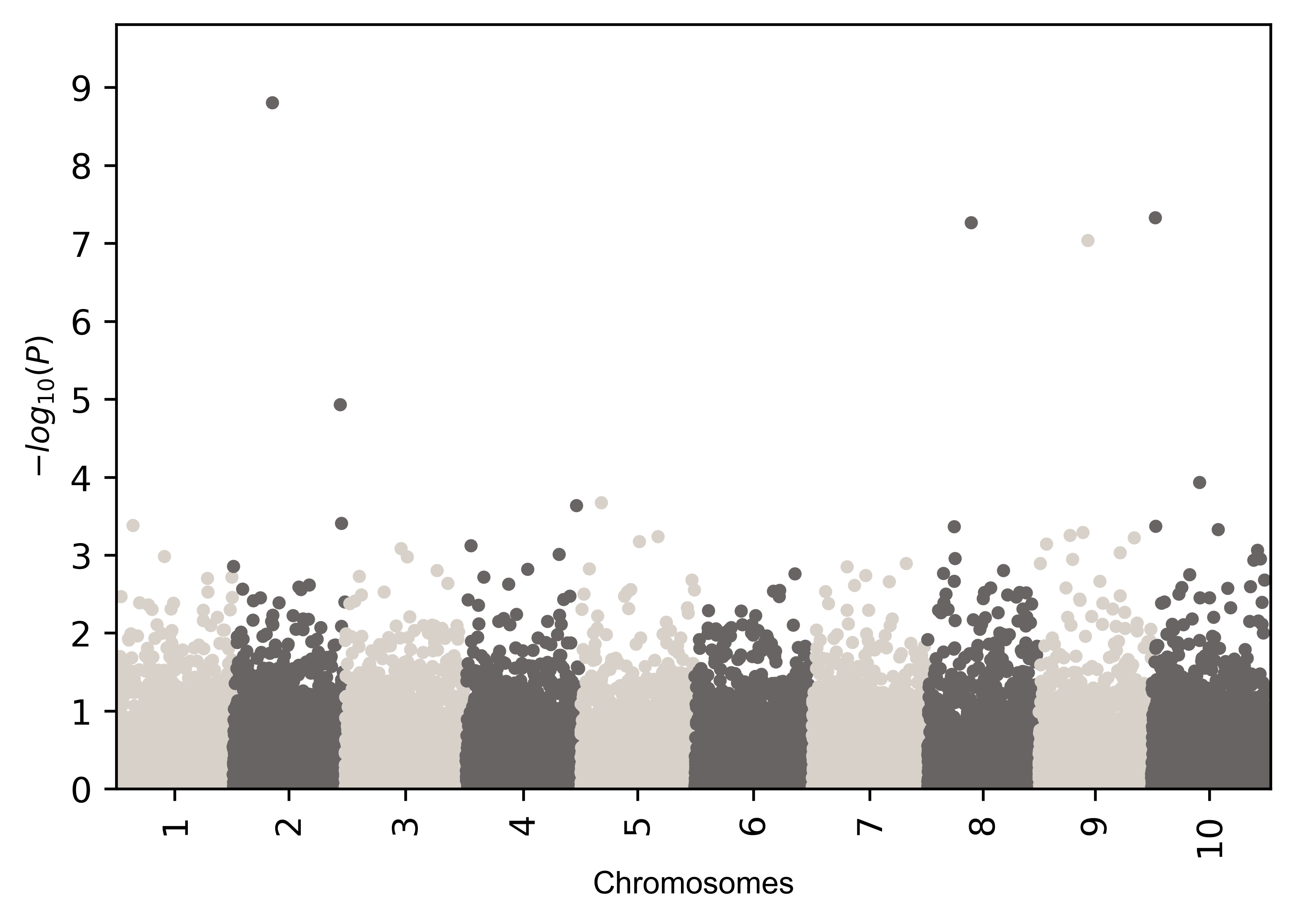``````# add different colors equal to number of chromosomes
color=("#a7414a", "#696464", "#00743f", "#563838", "#6a8a82", "#a37c27", "#5edfff", "#282726", "#c0334d", "#c9753d")
>>> visuz.marker.mhat(df=df, chr='chr',pv='pvalue', color=color)
``````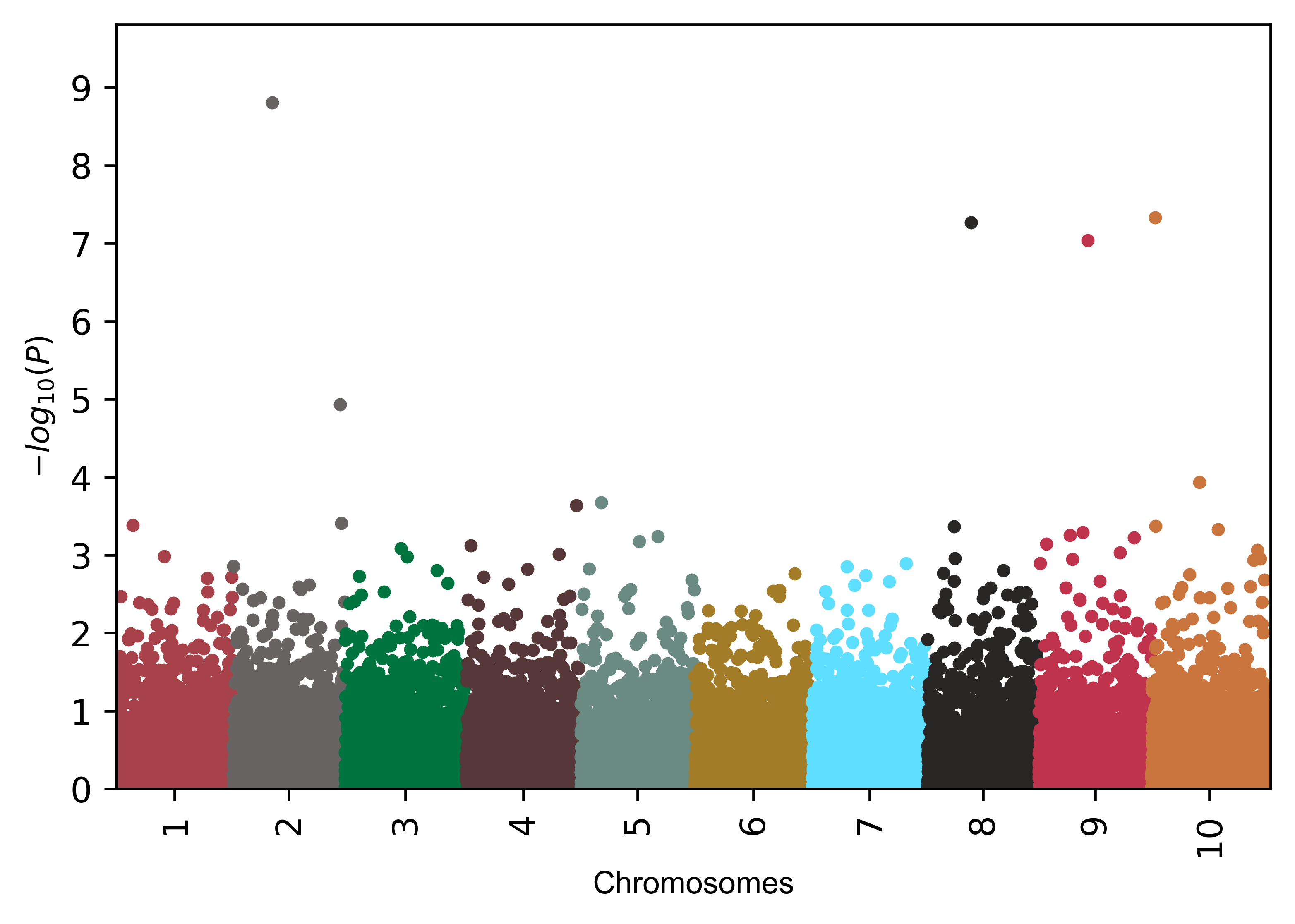``````# by default line will be plotted at P=5E-08
# you can change this value as per need
>>> visuz.marker.mhat(df=df, chr='chr',pv='pvalue', color=color, gwas_sign_line=True)
````````````# Change the position of genome-wide significance line
# you can change this value as per need
>>> visuz.marker.mhat(df=df, chr='chr',pv='pvalue', color=color, gwas_sign_line=True, gwasp=5E-06)
``````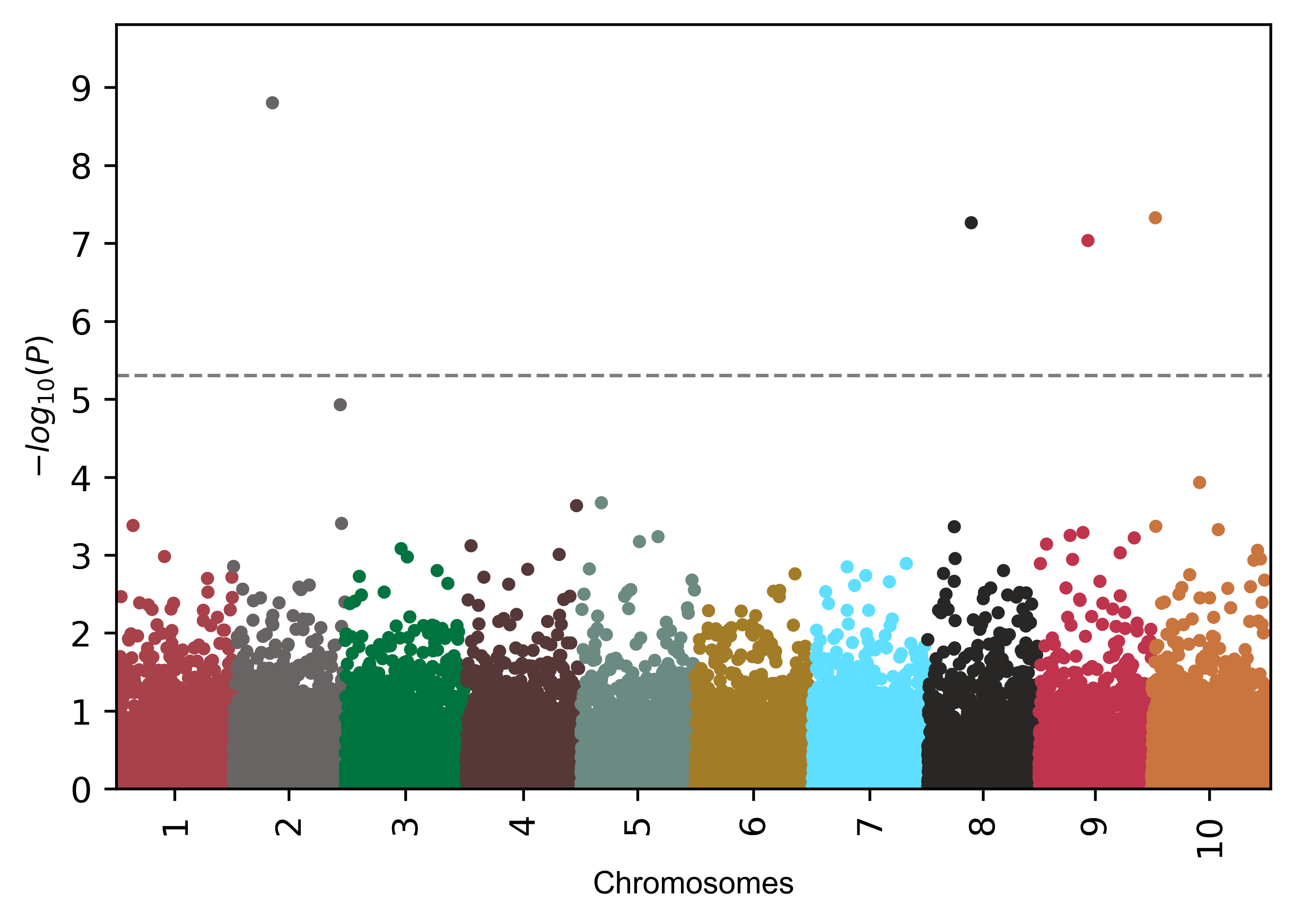Add annotation to SNPs (default text),

``````# add name to SNPs based on the significance defined by 'gwasp'
>>> visuz.marker.mhat(df=df, chr='chr',pv='pvalue', color=color, gwas_sign_line=True, gwasp=5E-06,
markernames=True, markeridcol='SNP')
``````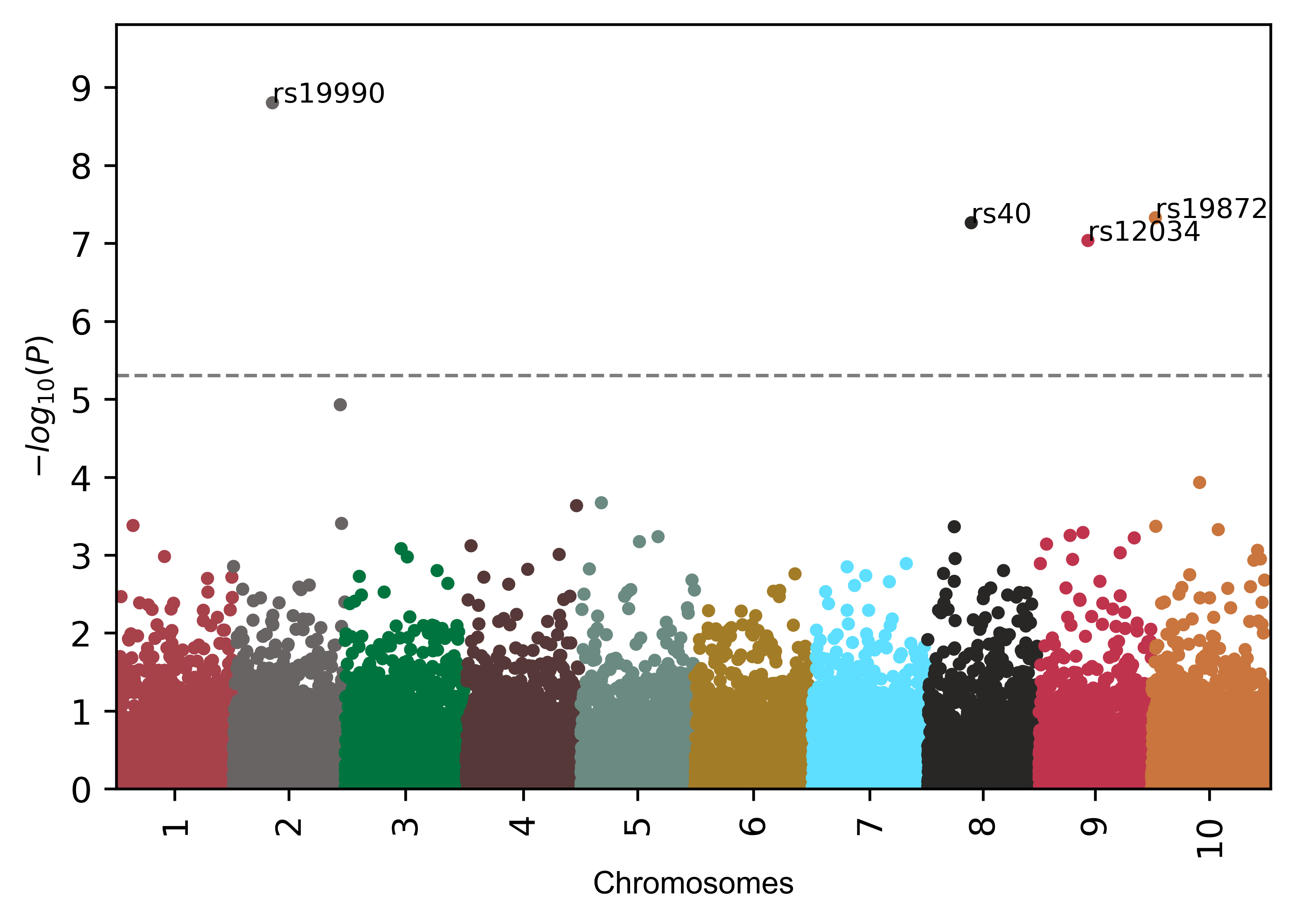Add annotation to SNPs (box text),

``````# add name to SNPs based on the significance defined by 'gwasp'
>>> visuz.marker.mhat(df=df, chr='chr',pv='pvalue', color=color, gwas_sign_line=True, gwasp=5E-06,
markernames=True, markeridcol='SNP', gstyle=2)
````````````# add name to specified  SNPs only
>>> visuz.marker.mhat(df=df, chr='chr',pv='pvalue', color=color, gwas_sign_line=True, gwasp=5E-06,
markernames=("rs19990", "rs40"), markeridcol='SNP')
``````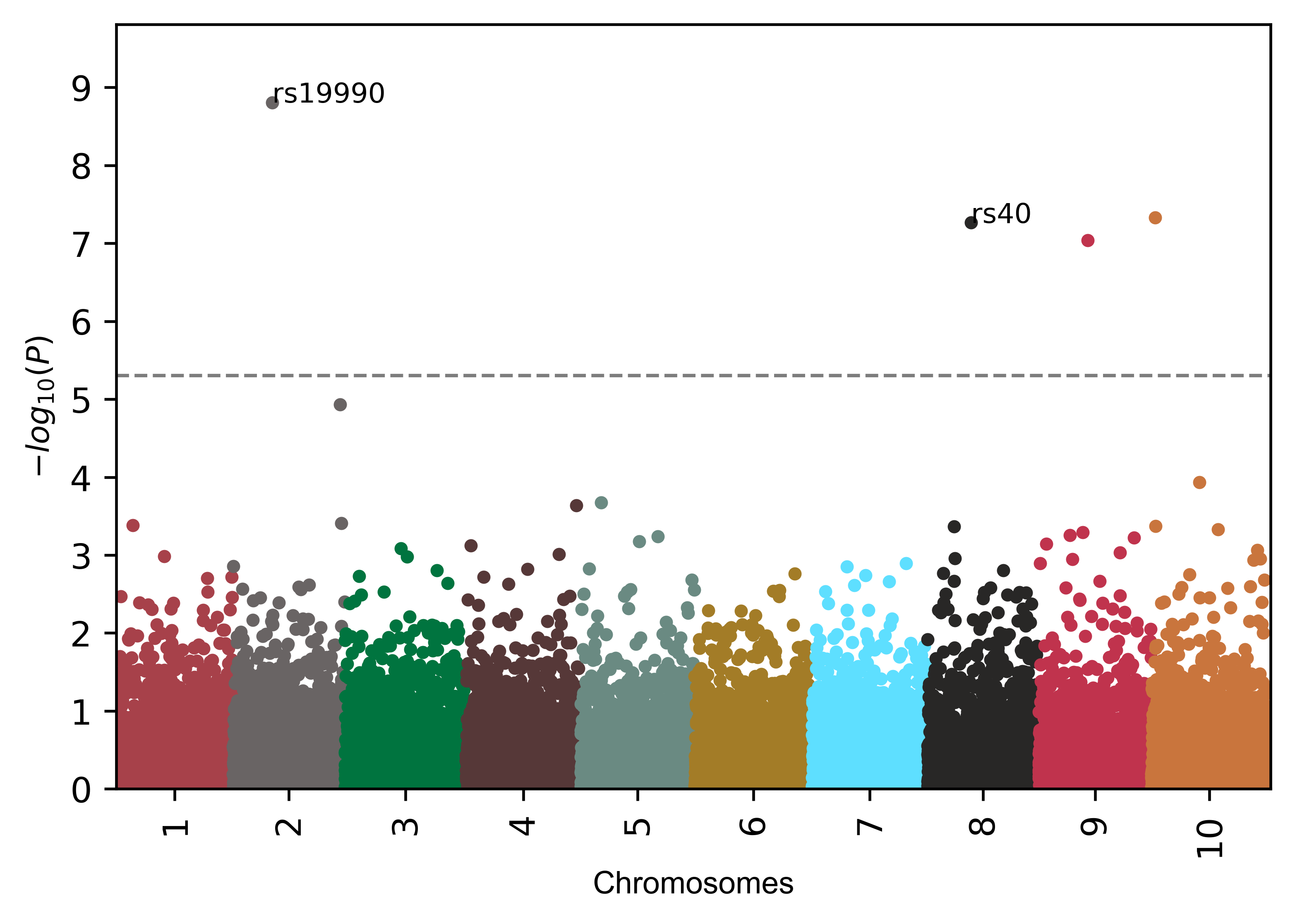``````# add name to specified  SNPs only (box text)
>>> visuz.marker.mhat(df=df, chr='chr',pv='pvalue', color=color, gwas_sign_line=True, gwasp=5E-06,
markernames=("rs19990", "rs40"), markeridcol='SNP', gstyle=2)
````````````# change fontsize of SNP annotation
>>> visuz.marker.mhat(df=df, chr='chr',pv='pvalue', color=color, gwas_sign_line=True, gwasp=5E-06, markernames=True,
markeridcol='SNP', gfont=5)
# gfont is incompatible with gstyle
````````````# add gene names to SNPs
>>> visuz.marker.mhat(df=df, chr='chr',pv='pvalue', color=color,
gwas_sign_line=True, gwasp=5E-06, markernames=({"rs19990":"gene1", "rs40":"gene2"}), markeridcol='SNP')
``````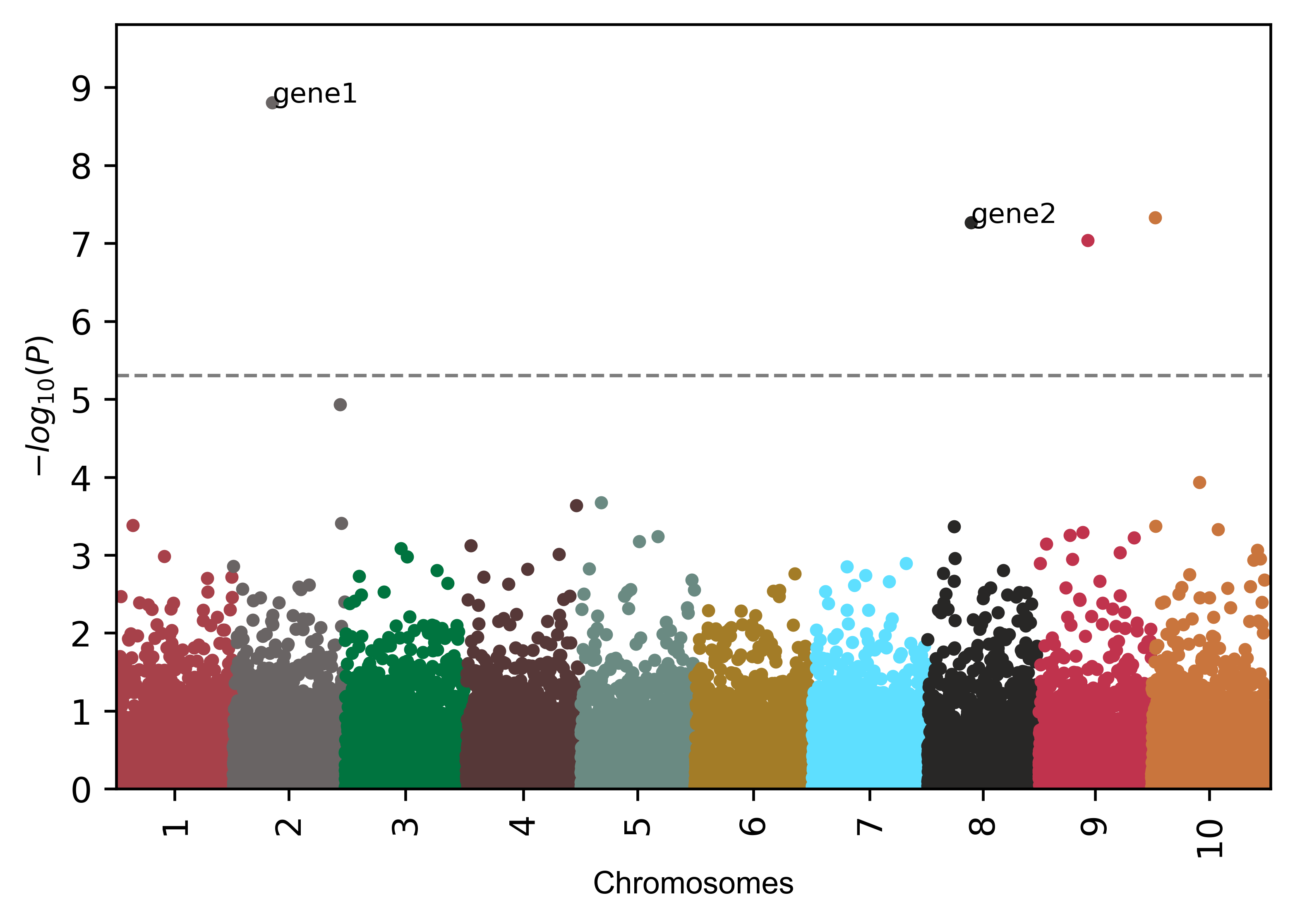Change fontsize, figure size, resolution, point size, and transparency

``````# change figure size
>>> visuz.marker.mhat(df=df, chr='chr',pv='pvalue', color=color, dim=(8,6) )
``````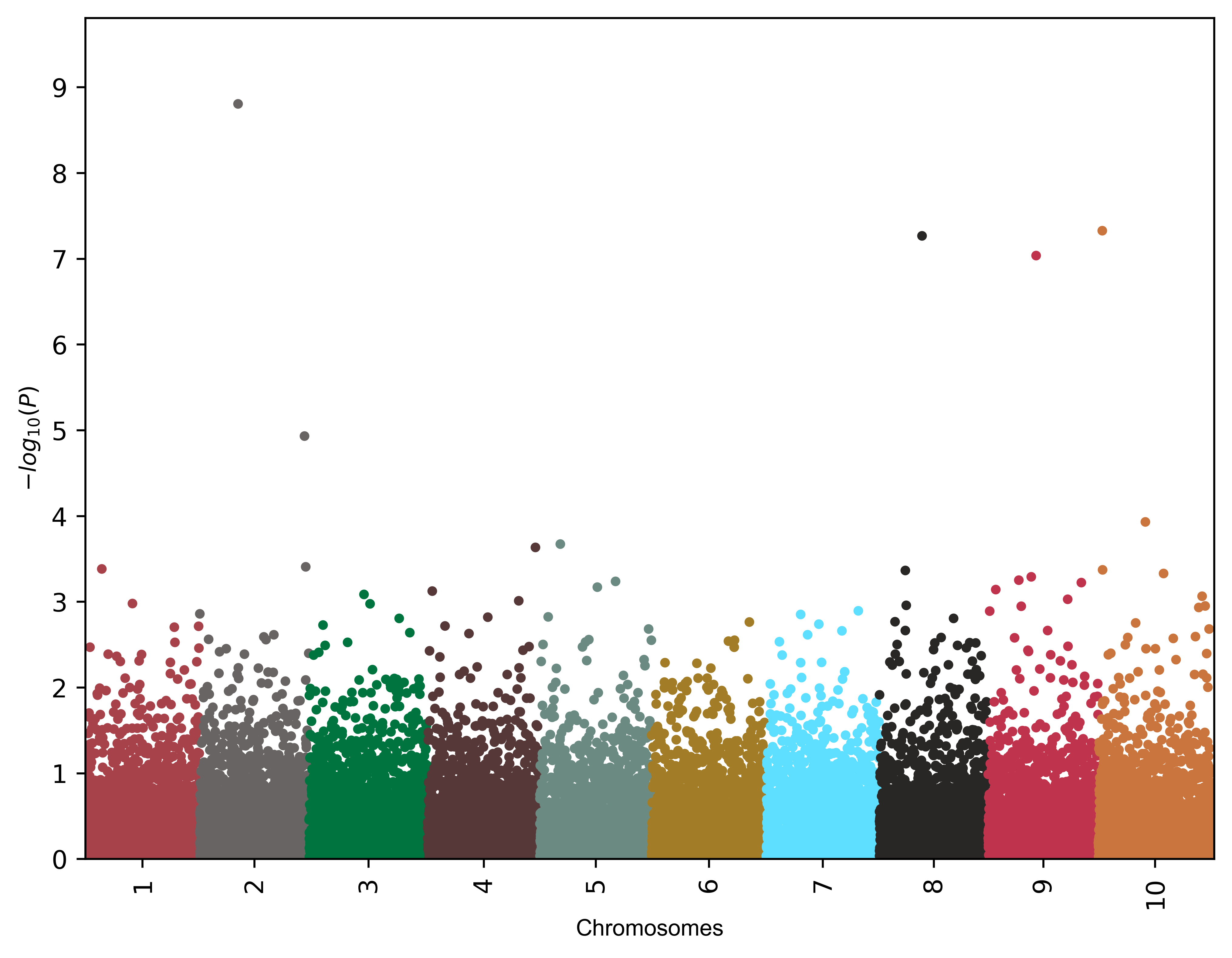``````# change point size
>>> visuz.marker.mhat(df=df, chr='chr',pv='pvalue', color=color, dotsize=2 )
``````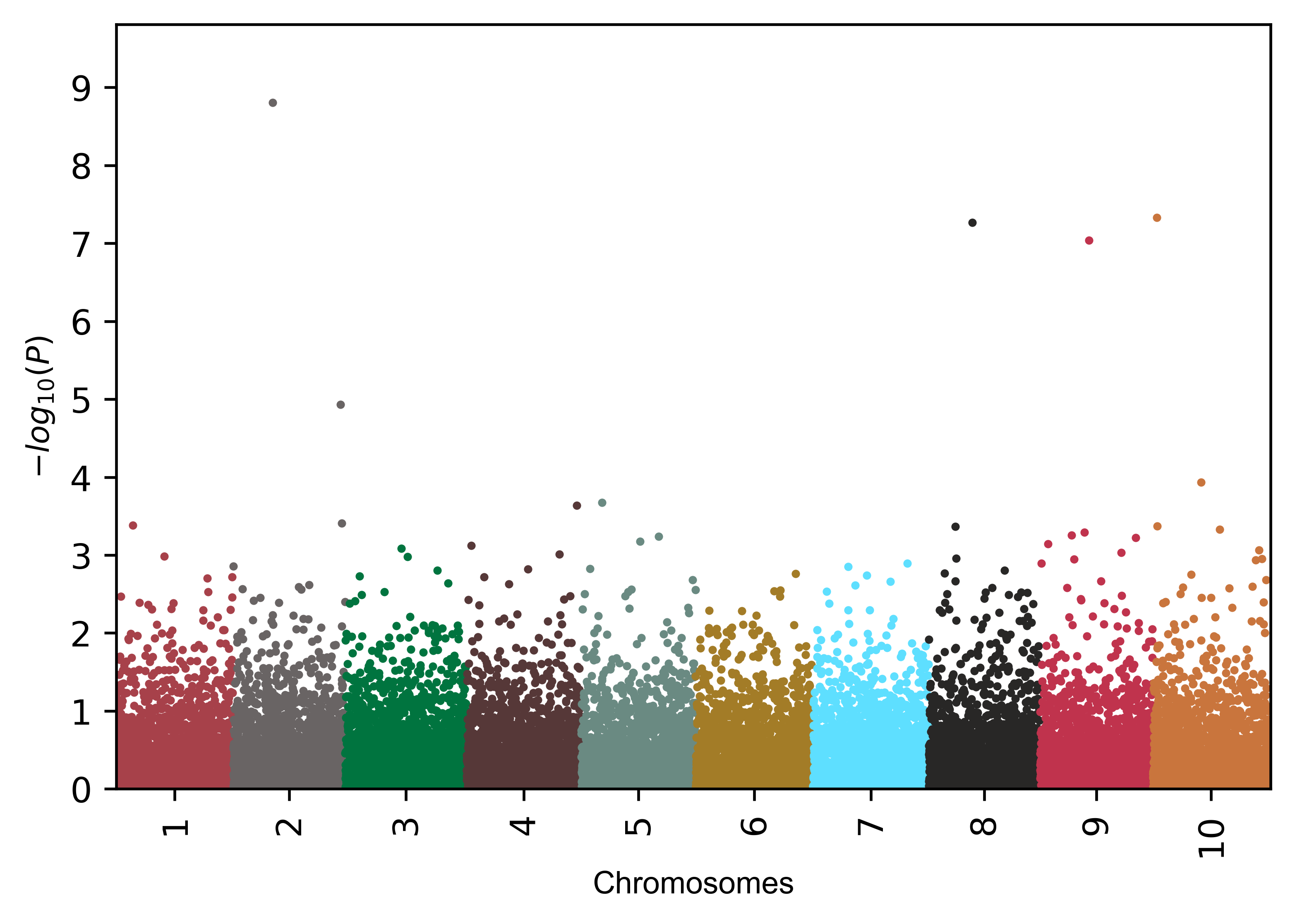``````# change point transparency
>>> visuz.marker.mhat(df=df, chr='chr',pv='pvalue', color=color, valpha=0.2 )
``````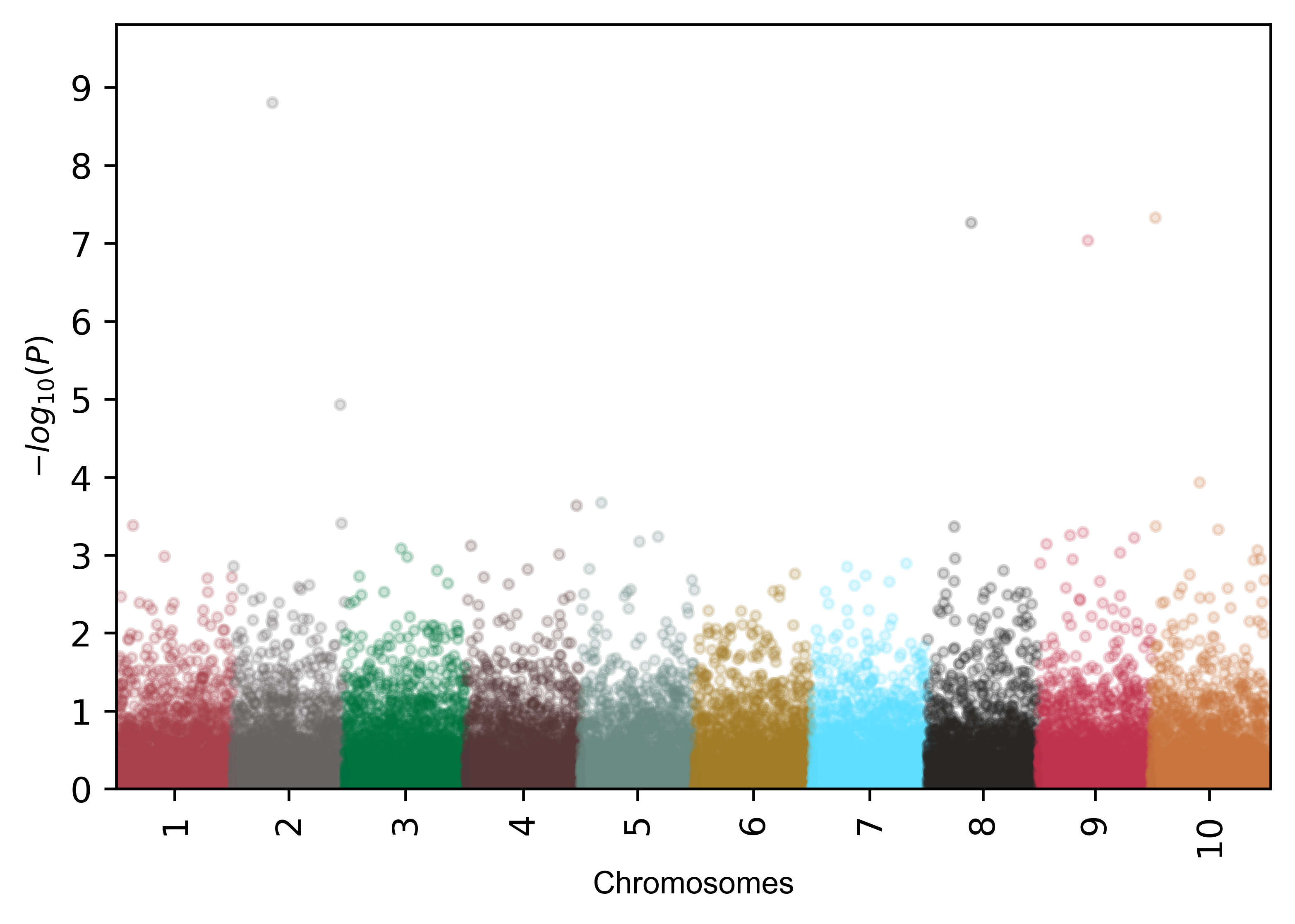``````# change X-axis tick label rotation
>>> visuz.marker.mhat(df=df, chr='chr',pv='pvalue', color=color, ar=60 )
``````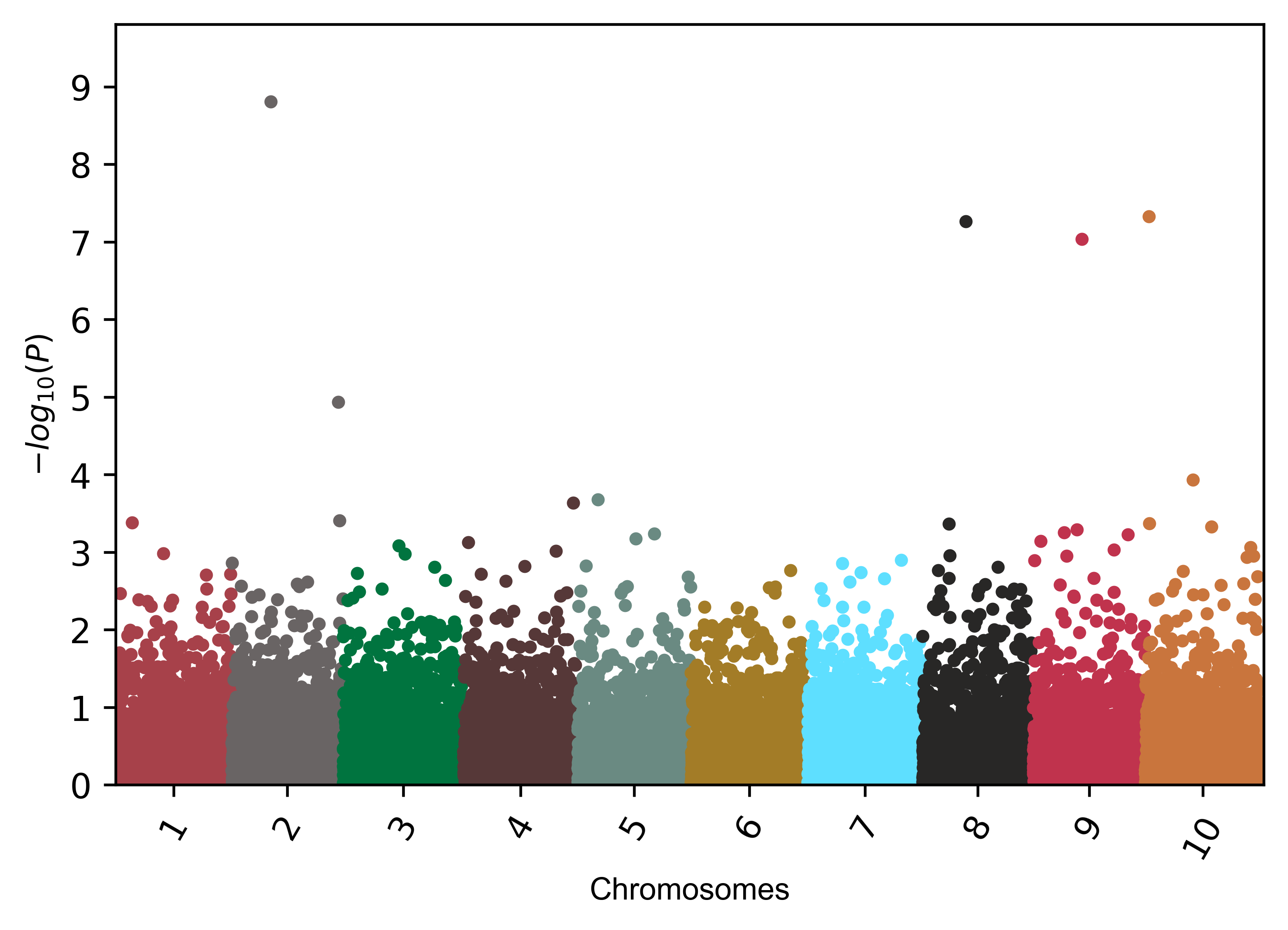``````# change figure resolution
>>> visuz.marker.mhat(df=df, chr='chr',pv='pvalue', color=color, r=600 )
``````In addition to these parameters, the parameters for figure type (`figtype`), Y axis ticks range (`ylm`), axis labels (`axxlabel`, `axylabel`),
axis labels font size (`axlabelfontsize``) can be provided.

Check detailed usage

How to cite?
Renesh Bedre.(2020, July 29). reneshbedre/bioinfokit: Bioinformatics data analysis and visualization toolkit (Version v0.9). Zenodo. http://doi.org/10.5281/zenodo.3965241

If you have any questions, comments or recommendations, please email me at reneshbe@gmail.com

Last updated: July 30, 2020

Tags: